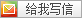# 1901: Zju2112 Dynamic Rankings

Seter posted @ 2012年2月01日 13:16 in BZOJ with tags ChairTree SegTree , 23708 阅读
 RunID User Problem Result Memory Time Language Code Length Submit Time 197772 Seter 1901 Accepted 31456 kb 380 ms C/Edit 2168 B 2012-02-01 11:05:39

#include <stdio.h>
#include <stdlib.h>
#define S struct Seg
#define mid (l+r>>1)
char*sp,str,*p=str;
int n,m,a,s,ra,K,Y=1,I,b,v={-2,-2};
S{S*c;int s}*R,st,*stp=st,*T;
getint(){
int re=0;
while(*p<'0'||*p>'9')p++;
while(*p>='0'&&*p<='9')re=re*10+*p++-48;
return re;
}cmp(const void*a,const void*b){return*(int*)b-*(int*)a;}
S*Build(l,r){
S*node=stp++;
if(l+1!=r)node->c=Build(l,mid),node->c=Build(mid,r);
return node;
}S*SegInsert(x,l,r)S*x;int l,r;{
S*node=stp++;node->s=x->s+Y;
if(l+1!=r){
if(I<mid)node->c=x->c,node->c=SegInsert(x->c,l,mid);
else node->c=x->c,node->c=SegInsert(x->c,mid,r);
}return node;
}void BitInsert(x){for(;x<=n;x+=x&-x)R[x]=SegInsert(R[x],0,K);}
void Init(x){for(;x;x-=x&-x)if(v[x]!=m)v[x]=m,T[x]=R[x];else v[x]=-2;}
void Turn(int x,_Bool c){for(;x;x-=x&-x)if(v[x]==m)T[x]=T[x]->c[c];}
BitQuery(x){int ans=0;for(;x;x-=x&-x)if(v[x]==m)ans+=T[x]->c->s;return ans;}
void print(x){
char out,*buf=out;
if(!x)*sp++='0';
else{
while(x)*buf++=x%10+48,x/=10;
while(buf--!=out)*sp++=*buf;
}*sp++='\n';
}main(){
for(s=n=i=getint(),m=getint();i--;a[i]=n-i-1)a[i]=getint();
for(sp=p;*p;)if(*p++=='C')getint(),a[s]=s,a[s++]=getint();
a[a[s]=s]=ra[s]=-1;
for(qsort(a,s,8,cmp);s--;b[ra[a[s]]=ra[a[s+1]]+(a[s]!=a[s+1])]=a[s]);
for(R[i=1]=Build(0,K=ra[a]+1);i++!=n;v[i]=-2)R[i]=R;
for(s=n,p=sp,sp=str,i=-1;++i!=n;BitInsert(i+1))I=ra[i];
while(m--){
while(*p!='Q'&&*p!='C')p++;
if(*p=='Q'){
i=getint()-1;j=getint();k=getint();
Init(i);Init(j);l=0;r=K;
while(l+1!=r)if((re=BitQuery(j)-BitQuery(i))>=k)Turn(i,0),Turn(j,0),r=mid;
else Turn(i,1),Turn(j,1),k-=re,l=mid;
print(b[l]);
}else if(ra[(i=getint())-1]!=ra[s++]){
I=ra[i-1];Y=-1;BitInsert(i);
I=ra[i-1]=ra[s-1];Y=1;BitInsert(i);
}
}fwrite(str,1,sp-str,stdout);return 0;
}By Seter
• 无匹配fotile 说:
2012年2月01日 13:51roosephu 说:
2012年2月21日 15:15roosephu 说:
2012年3月01日 22:19

Seter 说:
2012年3月02日 18:52nkuflk 说:
2012年7月24日 17:12

YM大神，这么神的东西。。就是排版看着真费劲。。yohanlong 说:
2016年7月07日 21:08

ym(输入验证码)
or Ctrl+Enter
Host by is-Programmer.com | Power by Chito 1.3.3 beta | © 2007 LinuxGem | Design by Matthew "Agent Spork" McGee